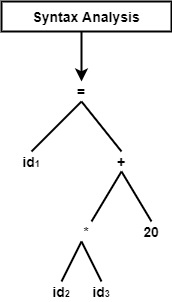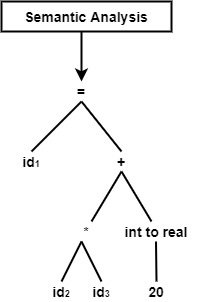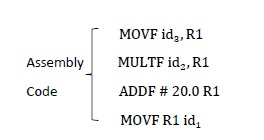# Show that the whole compilation process for statement A = B * C + 20, where A, B, C are of real types

Solution

Symbol Table

S.NO.Variable NameVariable Type
200AFloat
204BFloat
208CFloat

Now, we will see how we can perform the compiler phase at each level and how it works.

• Lexical Analysis

This is the first step that works as an integration between the compiler and the source language code. It reads the source code one character at a time and designs a source code into a series of atomic units known as tokens.

In this phase, we will see how we can tokenize the expression.

A → Identifier: (id, 1)

= → Operator: Assignment

B → Identifier: (id, 2)

* → Operator: Multiplication

C → Identifier: (id, 3)

20 → Constant: Integer

The final expression is as follows −

id1=id2*id3+20
• Syntax Analysis

It is also known as the parser. It receives tokens as its input generated from the previous phase (lexical analysis) and produces a hierarchical structure called syntax tree or parse tree.

In this phase, it can check the syntax after tokenized the expression.

Syntax Analysis for the expression is as follows −• Semantic Analysis

This phase makes the syntax tree input and determines the semantical accuracy of the program. However, the tokens are accurate and syntactically right; they may be precise, not semantically. Hence the semantic analyzer determines the semantics (meaning) of the statements construct. In this phase, it can verify the type and semantic action for the syntax tree.• Intermediate Code Generation

This phase takes the syntactically and semantically correct form as input and produces the same intermediate notation of the source code. In this phase, we will provide a changed parse tree and as output after transforming into the Intermediate program will create a three-address code.

T1=id2 * id3
T2=int to real (20)
T3=T1+T2
id1=T3
• Code Optimization

It is an optional phase. It converts the intermediate representation of the source program into an efficient code. In this phase, it would look as an input will provide three address code and as an output, and it will identify the optimized code.

T1=id2 * id3
id1=T1+ 20.0
• Code Generation

This is the final step of the compilation process. It converts optimized intermediate code into Machine/Assembly code. It allocates memory locations for variables in the program.

In the last phase, it can see how can modify the final expression into the assembly program.